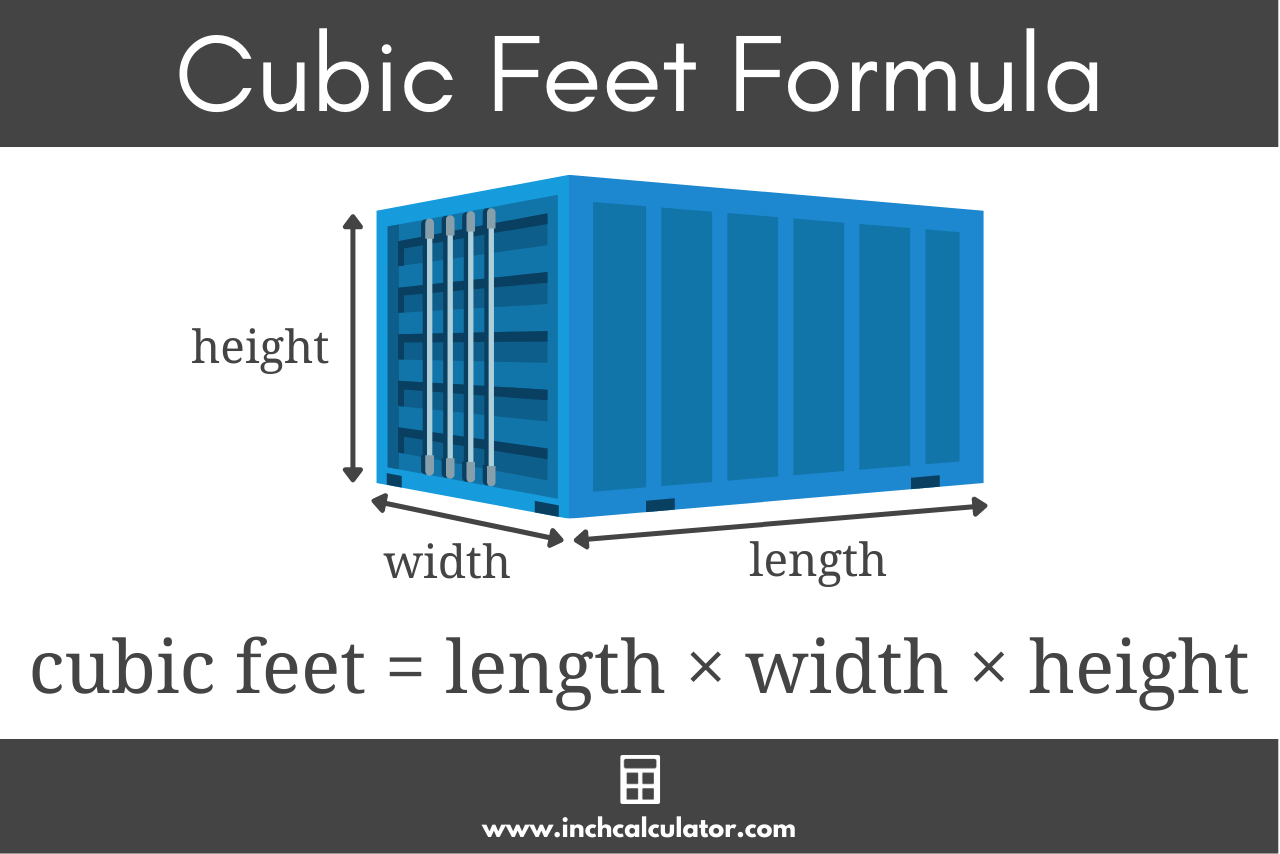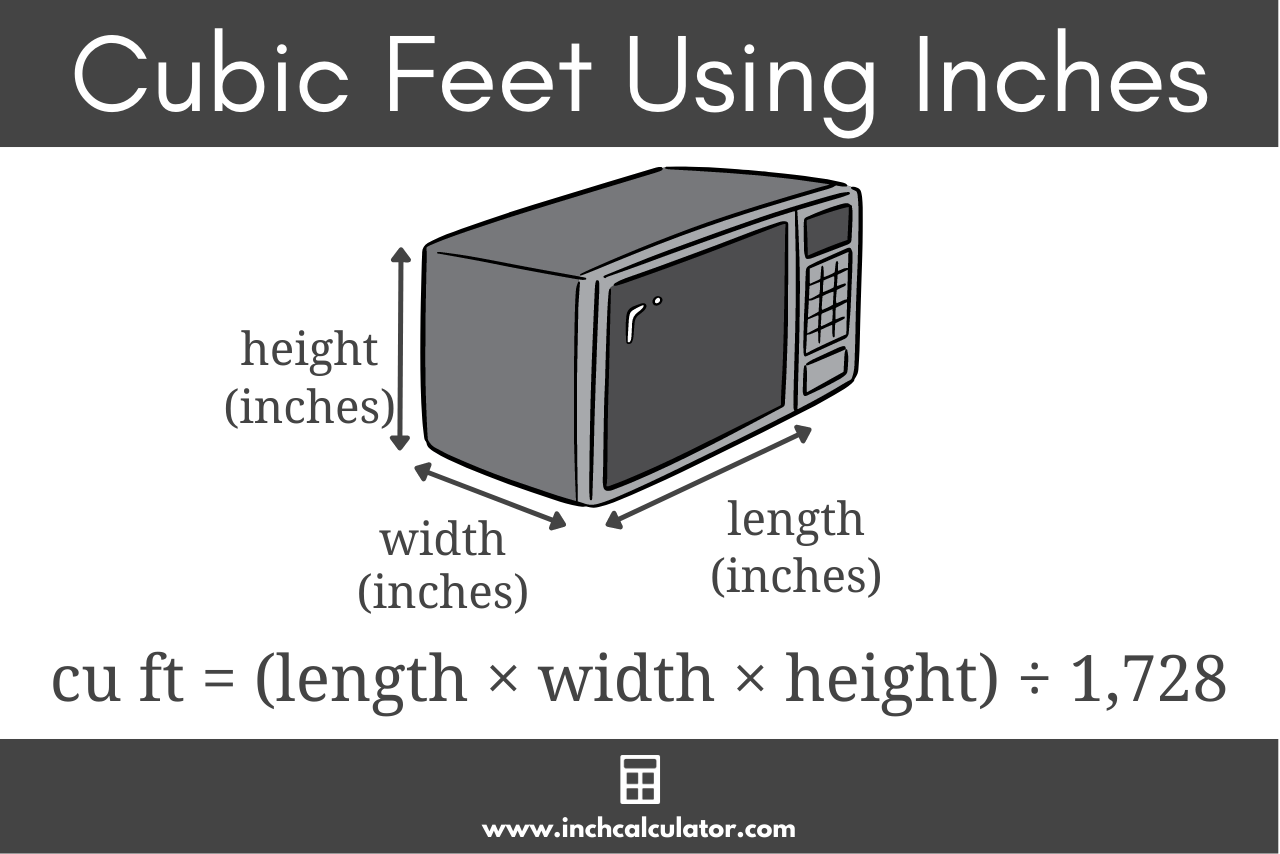# Cubic Feet Calculator

Calculate the cubic footage of a volume or space by entering the dimensions below. Enter dimensions in feet, inches, or even metric.

Optionally enter the price per cubic foot
\$
Optionally enter the price per cubic foot
\$

## Results:

Cubic Feet
Estimated Price
\$
Total Cost
Learn how we calculated this below

## How to Calculate Cubic Feet

Cubic footage is the volume of a space measured in feet. Knowing the cubic footage of an object or space is often necessary for shipping, freight, landscaping projects, and even when selecting a refrigerator or microwave.

The first step to finding the volume of an object or space in cubic feet is to measure the length, width, and height of a space or item.

Then, if the measurements were recorded using a different unit of length, convert them to feet.

Finally, apply the volume formula below to calculate the cubic footage.

### Cubic Footage Formula

To find the volume in cubic feet, multiply the three measurements in feet together. The volume formula looks like this:

volume = length × width × height

Thus, the volume in cubic feet is equal to the length times the width times the height, all measured in feet.For example, let’s calculate the cubic footage of a 10×20 storage unit that measures 20 feet long by 10 feet wide, and is 8 feet high.

volume = 20′ × 10′ × 8′
volume = 1,600 cu ft

So, a 10×20 storage unit has 1,600 cubic feet of space.

This calculator supports calculating the volume of a cube or rectangle; check out our volume calculator to find the volume of even more shapes.

### How to Calculate Cubic Footage Using Dimensions in Inches

If your measurements are in inches, then you can enter the inch measurements into the volume formula above and solve to find the volume in cubic inches.

Then, convert the volume in cubic inches to cubic feet by dividing the volume by 1,728. The number 1,728 comes from the fact that you have to divide by 12 inches per foot three times, one for each dimension.

Therefore, to obtain the volume in cubic feet, you can either divide the volume, in cubic inches, by 12 three times, or just divide it by 1,728, which is equal to 123.

cu ft = cu in ÷ 1,728

You can also convert using our handy cubic inches to cubic feet conversion utility.For example, let’s calculate the cubic feet of a microwave with a cooking area dimensions of 19″ by 14″ by 10″. Note that the volume of appliances are often measured using the interior dimensions rather than the exterior.

Start by finding the volume in cubic inches.

volume = 19″ × 14″ × 10″
volume = 2,660 cu in

Then divide by 1,728 to find cubic feet.

volume = 2,660 cu in ÷ 1,728
volume = 1.54 cu ft

So, this microwave has 1.54 cubic feet of cooking space.

## How to Find Cubic Feet Using Square Feet

Given the area of a space, it’s possible to find the volume in cubic feet if its height is also known. The formula for volume using area and height is:

volume = area × height

The volume is equal to the area times the height.

Since square footage is a measure of area, you can simply multiply the area in square feet times the height in feet to find the cubic footage.

For example, let’s calculate how many cubic feet of mulch you need to fill a 50 square foot area with 6″ of mulch. Note that 6″ is equal to 1/2 a foot.

volume = 50 sq ft × 1/2 ft
volume = 25 cu ft

So, in this example you’ll need 25 cubic feet of mulch for this landscaping project. This is the same formula our mulch calculator uses.

You can also use our square footage to cubic footage calculator to simplify this calculation.

## How to Convert a Volume to Cubic Feet

If you have a volume measurement in cubic inches, cubic yards, cubic centimeters, or cubic meters, you can convert it to cubic feet using the appropriate formula below.

This table shows the formula to convert several forms of volume to cubic footage.
Volume Unit Formula
cubic inches cu ft = cu in ÷ 1,728
cubic yards cu ft = cu yds × 27
cubic centimeters cu ft = cu cm ÷ 28,316.8
cubic meters cu ft = cu m × 35.3

For example, let’s convert 3 cubic yards to cubic feet.

Start by locating the formula in the table above.

volume = cu yds × 27

Then enter the measurement into the formula and solve.

volume = 3 × 27
volume = 81 cu ft

You can also use a volume conversion calculator to easily convert between different volumetric units.

### What is a cubic foot?

A cubic foot is a measurement of the volume of a space in feet. In other words, cubic footage is the volume of a space calculated from its dimensions measured in feet.

One cubic foot is equivalent to the volume of a cube with width, length, and height equal to one foot.Measurements in cubic feet are primarily used in the US and parts of Canada. The rest of the world has adopted the metric system and typically uses metric volume measurements such as cubic meters.

### How big is a cubic foot?

As mentioned above, a cube with a volume of one cubic foot has sides that are one foot in each dimension. See the table below to see how big a cubic foot is in other units of measure.

Dimensions of a cube with a volume of one cubic foot.
UnitDimensions
inches12″ × 12″ × 12″
yards0.334 yd × 0.334 yd × 0.334 yd
centimeters30.48 cm × 30.48 cm × 30.48 cm
meters0.3048 m × 0.3048 m × 0.3048 m

### How do you calculate cubic feet using dimensions in other units?

If your measurements are in yards, centimeters, meters, or another unit of length, then the process of finding cubic footage is similar to that when the dimensions are in inches.

Start by converting your measurements to feet. You can use a length converter to convert to feet, and the calculator above allows you to enter different units as well.

Finally, enter the converted measurements into the volume formula and solve.

### How do you find the cubic footage of a cylinder?

To find the volume of a cylinder, use this formula:

cu ft = π × radius2 × height

Enter the radius and height in feet to get the volume in cubic feet. We also have a cylinder volume calculator that makes it easy to calculate.

### Are cubic feet the same as ft3?

Yes! Cubic feet can be expressed as ft3, cu ft, or CF, so you might see 5 cubic feet written as 5 ft3, 5 cu ft, or 5 CF.

### How many cubic feet is 3' x 3' x 3'?

A 3′ x 3′ x 3′ space is equal to 27 cubic feet, which is also equal to 1 cubic yard.

3 ft × 3 ft × 3 ft = 27 cu ft

## References

1. Approved Freight Forwarders, How to Calculate Cubic Feet & Other Easy Freight Math, https://www.approvedforwarders.com/how-to-calculate-cubic-feet/
2. Wikipedia, Cubic foot, https://en.wikipedia.org/wiki/Cubic_foot